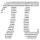# Perimeter - 8th grade (13y) - examples

1. Diamond and diagonalsA diamond has diagonals f = 8 cm and g = 6 cm long. How long is this diamond perimeter? (Calculate it!)
2. The sides 2The sides of a trapezoid are in the ratio 2:5:8:5. The trapezoid’s area is 245. Find the height and the perimeter of the trapezoid.
3. GardenArea of square garden is 6/4 of triangle garden with sides 56 m, 35 m and 35 m. How many meters of fencing need to fence a square garden?
4. Triangle SASCalculate area and perimeter of the triangle, if the two sides are 51 cm and 110 cm long and angle them clamped is 130°.
5. RhombusCalculate the perimeter and area of ​​rhombus whose diagonals are 38 cm and 55 cm long.
6. Right triangle AlefThe obvod of a right triangle is 84 cm, the hypotenuse is 37 cm long. Determine the lengths of the legs.
7. RectangleIn rectangle with sides 10 and 8 mark the diagonal. What is the probability that a randomly selected point within the rectangle is closer to the diagonal than to any side of the rectangle?
8. CylindersArea of the side of two cylinders is same rectangle of 50 cm × 11 cm. Which cylinder has a larger volume and by how much?
9. Circle arcCircle segment has a circumference of 72.95 cm and 1386.14 cm2 area. Calculate the radius of the circle and size of central angle.
10. Square diagonalCalculate length of the square diagonal if the perimeter is 476 cm.
11. StepsHow many steps you save if you go square estate for diagonal (crosswise), rather than circumvent on the two sides of its perimeter with 307 steps.
12. Rectangle - sides ratioCalculate area of rectangle whose sides are in ratio 3:13 and perimeter is 673.
13. Isosceles right triangleCalculate the area of an isosceles right triangle whose perimeter is 377 cm.
14. ConeCircular cone of height 15 cm and volume 5699 cm3 is at one-third of the height (measured from the bottom) cut by a plane parallel to the base. Calculate the radius and circumference of the circular cut.
15. RadiansConvert 270° to radians. Write result as multiple of number π.
16. ArcCircle arc corresponding to angle is 32° is 28 dm long. What is the length of the entire circle?
17. DescribedCalculate perimeter of the circle described by a triangle with sides 478, 255, 352.
18. R TrapeziumRectangular trapezium has bases 12 and 5 and area 84 cm2. What is its perimeter?
19. Arc and segmentCalculate the length of circular arc l, area of the circular arc S1 and area of circular segment S2. Radius of the circle is 33 and corresponding angle is ?.
20. AnglesDetermine the interior angles of a rhombus with area 319.1 cm2 and perimeter 72 cm.

Do you have an interesting mathematical example that you can't solve it? Enter it, and we can try to solve it.

To this e-mail address, we will reply solution; solved examples are also published here. Please enter e-mail correctly and check whether you don't have a full mailbox.